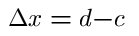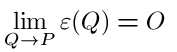#Linear Approximations

### Linear Approximations for one-variable functions

Lets say f(x) is a one-variable function. Now let P=(c,0) and Q=(d,0) be points on the x-axis, such that f is defined on both P and Q. Let

##So we can rewrite,

##is the change in the value of f from P to Q. Consider the very familiar quotient

##Taking the limit of this quotient as Q approaches P hearkens us back to the definition of taking of a derivative back in basic calculus. This limit, if it exists, gives us the derivative of f at c. In the infant years of calculus, this derivative was not thought of as the rate of change of f but of as a means of getting an approximate value for the change in f when c and the change in x are given. That is the formula,

##provides an approximate value for the change in f and is called the linear approximation formula for f at x=c. The constant f'(c) was called the differential coefficient of f at c.

Of course any approximation function also has its error function, which in this case is equal, for any point Q to,

##Let's formally define a linear approximation.
Definition: Let f(x) be a one-variable function on a domain D. Let x=c be in D and let P, Q the change in x be defined as above. We say f has a linear approximation at c if, for our chosen c, there is a constant A and an error function of Q, such that:

1. for all Q in D,

2. ##This definition implies

• if f has a linear approximation at c, then f is continuous at c.
• f has a linear approximation at c if and only if f has a derivative at c.

### Linear approximation for multi-variable functions

The definition for linear approximation for a multi-variable function will be (not surprisingly) similar to that of one variable.
Definition: Let f( x1,x2,...xn) be a n-variable function on domain D. Let P be a fixed point in D, P=(a1,a2,...an). Let Q=((a1+x1),(a2+x2),...(an+xn)) be another point in D. Letf = f((a1+x1),(a2+x2),...(an+xn))-f(a1,a2,...an). We say that f has a linear approximation at P if, for our chosen P, there exist constants A1,A2,...An and n functions erf1(Q), erf2(Q),... erfn(Q) such that

1. for all Q in D,f= A1x1 + A2x2+...Anxn+ erf1(Q)x1 + erf2(Q)x2 + ... +erfn(Q)xn
2. and as Q approaches P all the error functions of Q go to zero.

This definition implies the following:

• If f has linear approximations at P, then f is continuous at P
• if f has linear approximations at P, then f has first-order partial derivatives at P and these derivatives have the values of fx1(P)=A1 , fx2(P)=A2 , . . . fxn(P)=An

One must note that it is possible for f to have first-order partial derivatives at every point, but not to have linear approximations at a certain point P in its domain. That is, a f can have first-order partial derivatives at every point, but not be continuous at a certain point P in its domain.

Vector Calculus Index | World Web Math Main Page

thing@athena.mit.edu
Last modified 24 July 1997